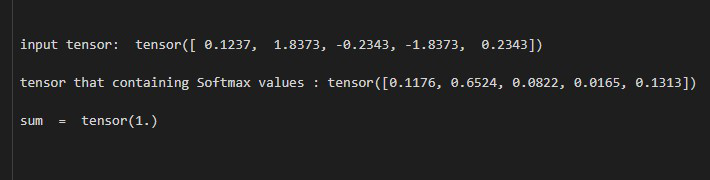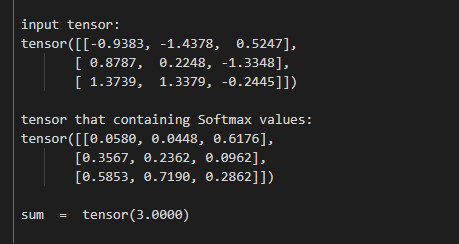Open in App
Not now

# How to Rescale a Tensor in the Range [0, 1] and Sum to 1 in PyTorch?

• Last Updated : 02 Jun, 2022

In this article, we are going to discuss How to Rescale a Tensor in the Range [0, 1] and Sum to 1 in PyTorch using Python.

## Softmax() method

The Softmax() method helps us to rescale a tensor of n-dimensional along a particular dimension, the elements of this input tensor are in between the range of [0,1] and the sum to 1. This method returns a tensor of the same shape and dimension as the input tensor and the values lie within the range [0, 1]. before moving further let’s see the syntax of the given method.

Syntax: torch.nn.Softmax(dim)

Parameters:

• dim: The dim is dimension in which we compute the Softmax.

Returns: It will returns a tensor with same shape and dimension as the input tensor and the values are in between the range [0, 1].

Example 1: In this example, we rescale a 1D tensor in the range [0, 1] and sum to 1.

## Python

 `# import required libraries ` `import` `torch ` ` `  `# define a tensor ` `input_tens ``=` `torch.tensor([``0.1237``, ``1.8373``,  ` `                           ``-``0.2343``, ``-``1.8373``, ` `                           ``0.2343``]) ` ` `  `print``(``" input tensor: "``, input_tens) ` ` `  `# Define the Softmax function ` `softmax ``=` `torch.nn.Softmax(dim``=``0``) ` ` `  `# Apply above defined Softmax function ` `# on input tensor ` `output ``=` `softmax(input_tens) ` ` `  `# display tensor that containing Softmax values ` `print``(``" tensor that containing Softmax values: "``,  ` `      ``output) ` ` `  `# display sum ` `print``(``" sum  = "``, output.``sum``()) `

Output:Example 2: In this example, we rescale a 2D tensor in the range [0, 1] and sum to 1.

## Python

 `# import required libraries ` `import` `torch ` ` `  `# define a tensor ` `input_tens ``=` `torch.tensor([[``-``0.9383``, ``-``1.4378``, ``0.5247``], ` `                           ``[``0.8787``,  ``0.2248``, ``-``1.3348``], ` `                           ``[``1.3739``,  ``1.3379``, ``-``0.2445``]]) ` ` `  `print``(``"\n input tensor: \n"``, input_tens) ` ` `  `# Define the Softmax function ` `softmax ``=` `torch.nn.Softmax(dim``=``0``) ` ` `  `# Apply above defined Softmax function on  ` `# input tensor ` `output ``=` `softmax(input_tens) ` ` `  `# display tensor that containing Softmax values ` `print``(``"\n tensor that containing Softmax values: \n"``, output) ` ` `  `# display sum ` `print``(``"\n sum  = "``, output.``sum``()) `

Output:My Personal Notes arrow_drop_up
Related Articles